# Manifolds

### Basic concepts and principles

Manifold concepts extends to regular surfaces and curves in R n. Intuitively, a manifold can be considered as a geometric object constructed from "parts of R n differentially glued." Where we mean by parts those open sets in the induced usual topology, will be discuss it in detail.

Imagine the simple pendulum.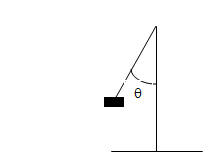to give the position of the pendulum is like to give the vertical angle θ formed with vertical, therefore θ is a number in the interval [0, 2π]. This is, a position in the circle that is usually denoted by S1 and the form is.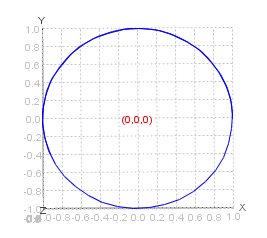now consider the double pendulum case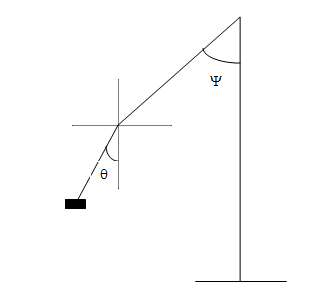Now to give the position of the pendulum at a given time, two numbers θ and Ψ in the interval [0,2π] are needed. So, the object we need is for both is those formed by cartesian product S1xS1 whose object is a geometric figure called Torus S1. It surface is like.If we up the number of dimensions, for example if we consider the triple pendulum, we need three numbers at [0,2π], The object is now S1xS1 xS1.

We can not draw that object because we'd need 4 dimensions for it(or in another way: the minimum n for which there immersion of this object in Rn is n=4), Yet we know that, those object contain a piece of R 3, because represent an infinitesimal motion of the 3 pendulums, the object you needed is similar to Cartesian product of 3 small intervals, or an infinitesimal cube.

### Definition of Chart and parameterization

Given a topological space M with the topology T, a chart is a pair (U, φ) such us U∈T and φ are Homeomorphism

φ: U⊂M → V⊂Rn being V=W∩φ(U)

with W, an open set of Rn. Ie φ(U) is open in the topology inherited from the image Rn.
The inverse of the charts ρ are called parametrizations.

ρ : φ-1(U)⊂Rn → U⊂M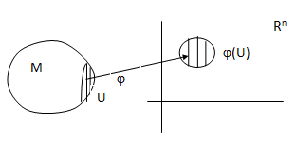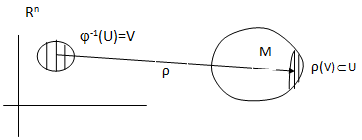Chart of a Manifold Parametrization of a Manifold

### Maximal (or complete) Atlas Definition

An Maximal (or complete) Atlas C on M is a chart collection (Uα, φα) such us

1) M⊂ ∪α Uα, ie Uα is a cover of M.

2) Lets W = φα(Uα) ∩ φβ (Uβ) with W≠ ∅, then, the apps
φαºφβ-1 : φβ(Uβ ) → φα(Uα)
φβºφα-1 : φα(Uα) → φβ(Uβ)
are C applications.

3) The atlas is maximal,it means that the atlas is not contained in another atlas, ie it contains all the possible charts.
We have all the ingredients for the definition of manifold, simply glue these pieces of Rn.

### Manifold Definition and the Tangent Space

A Manifold C is a Hausdorff topological space dotted with a C maximal atlas .

In a Curve in Rn tangent space is defined as that spanned by the vector tangent to the curve.

In a regular surface Rn tangent space is defined as that generated by two linearly independent tangent vectors of the surface.

In a general manifold there are not environment space, so concept of tangent space is defined in a way a little different. We take as tangent vectors, directional derivative operator in each environment of parametrization, defined by the direction for each coordinate curve, exactly, let x be a point on the manifold M, leta function corresponding to a coordinate environment containing x and let f be a function of the manifold in Rn, Then we define a tangent vector at x in the direction i asSo we consider the basis vectors formed by {,,...,} which generate a vector space at each point p of M, and we call the tangent space of M at p and denote by Tp(M). Therefore

Tp(M) = SPAN({,,...,})

At the same time, we define the dual of Tp(M), also called cotangent space at p and denoted as
Tp(M) * which is generated by the basis of covectors.

Tp(M)* = SPAN({dx1,..., dxn})

also called 1-forms.

# Was useful? want add anything?

Post here

### Post from other users

Post here